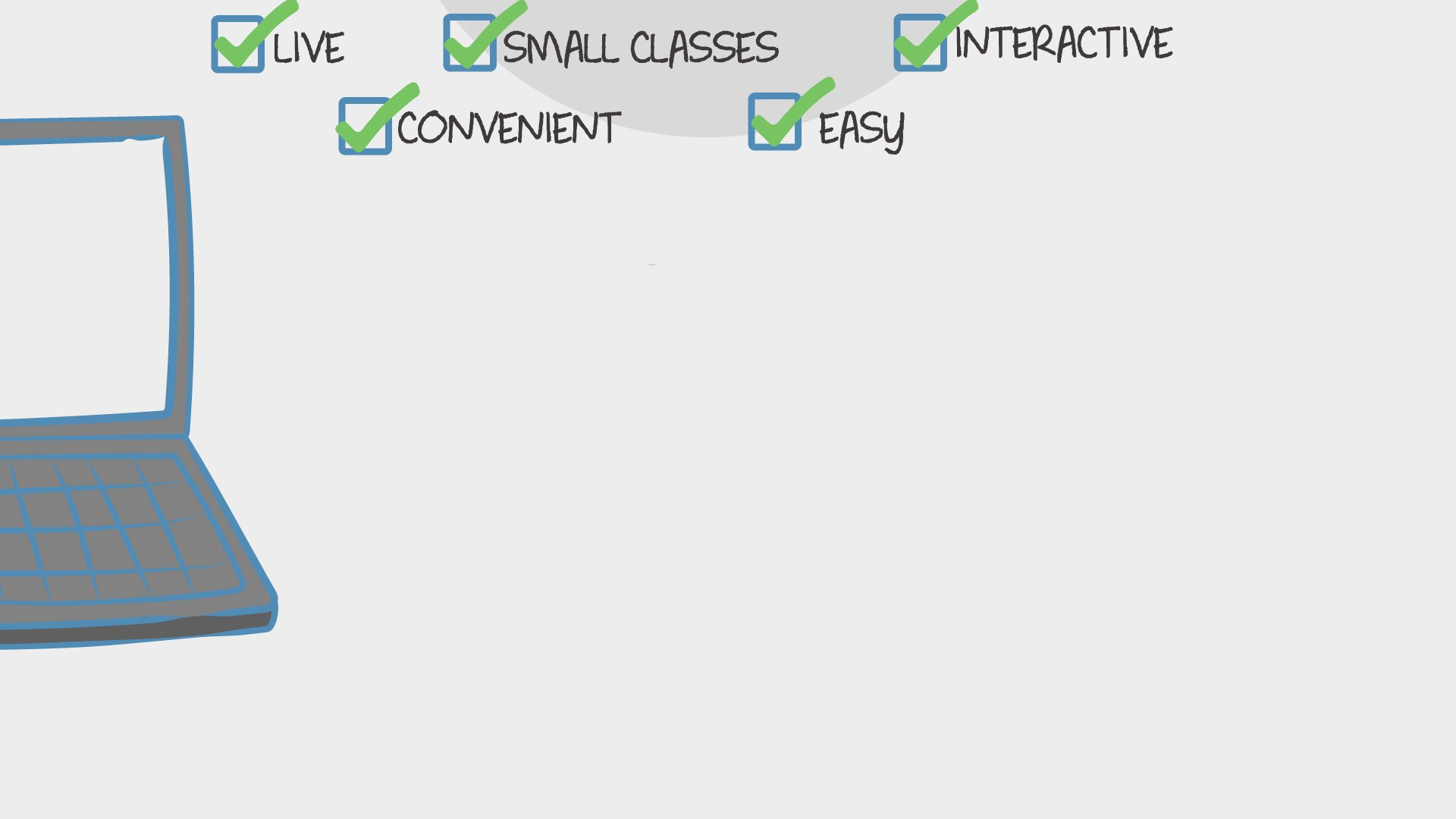Find Classes
Teach

Math

# 1st Grade Math (1st Quarter)

In this 8 week long class, we will meet all the common core math standards for the 1st quarter of 1st grade.550 total reviews for this teacher
3 reviews for this class
Completed by 6 learners
There are no upcoming classes.40 minutes
per class
Twice per week
over 8 weeks
5-8
year olds
2-6
learners per class
per learner - per class

### How does a “Multi-Day” course work?

Meets multiple times at scheduled times
Live video chats, recorded and monitored for safety and quality
Discussions via classroom forum and private messages with the teacher
Great for engaging projects and interacting with diverse classmates from other states and countries### How Outschool Works### There are no open spots for this class.

You can request another time or scroll down to find more classes like this.

## Description

### Class Experience

```1.OA.1 Use addition and subtraction within 20 to solve word problems involving situations of adding to, taking from, putting together, taking apart, and comparing, with unknowns in all positions, e.g., by using objects, drawings, and equations with a symbol for the unknown number to represent the problem.

1.OA.3 Apply properties of operations as strategies to add and subtract. (Students need not use formal terms for these properties.) Examples:  If 8 + 3 = 11 is known, then 3 + 8 = 11 is also known.  (Commutative property of addition.)  To add 2 + 6 + 4, the second two numbers can be added to make a ten, so 2 + 6 + 4 = 2 + 10 = 12. (Associative property of addition.)

1.OA.5 Relate counting to addition and subtraction (e.g., by counting on 2 to add 2).

1.OA.6 Add and subtract within 20, demonstrating fluency for addition and subtraction within 10.  Use strategies such as counting on; making ten (e.g., 8 + 6 = 8 + 2 + 4 = 10 + 4 = 14); decomposing a number leading to a ten (e.g., 13 – 4 = 13 – 3 – 1 = 10 – 1 = 9); using the relationship between addition and subtraction (e.g., knowing that 8 + 4 = 12, one knows  12 – 8 = 4); and creating equivalent but easier or known sums (e.g., adding 6 + 7 by creating the known equivalent 6 + 6 + 1 = 12 + 1 = 13).

1.OA.7 Understand the meaning of the equal sign, and determine if equations involving addition and subtraction are true or false.  For example, which of the following equations are true and which are false?  6 = 6, 7 = 8 – 1, 5 + 2 = 2 + 5, 4 + 1 = 5 + 2.

1.OA.8 Determine the unknown whole number in an addition or subtraction equation relating three whole numbers.  For example, determine the unknown number that makes the equation true in each of the equations 8 + ? = 11
I have a degree in elementary education with an emphasis in math and social science. I taught in a classroom for 8 years. I have taught both 1st and 2nd grade.
There will be extra activities that will be available for students to work on outside of the classroom. These are for extra practice and will not need to be turned in.
Students will need a paper and pencil for every class. Some classes they may need additional materials such as printed pages or manipulatives. Students will not need to buy anything for this class. The manipulatives needed with be things you can find around the house or I will provide a page that can be printed off.
Learners will not need to use any apps or websites beyond the standard Outschool tools.
I will assess student through observation. I will also provide assessments to parents if they would like to have a formal assessment for their learner.Parents can also use the extra practice pages to assess where their learner is at if they would like.
1 hour 15 minutes per week in class, and maybe some time outside of class.
```
`I have a degree in elementary education with an emphasis in math and social science. I taught in a classroom for 8 years. I have taught both 1st and 2nd grade and have also homeschooled. `
`There will be extra activities that will be available for students to work on outside of the classroom. These are for extra practice and will not need to be turned in. `
`Students will need a paper and pencil for every class. Some classes they may need additional materials such as printed pages or manipulatives. Students will not need to buy anything for this class. The manipulatives needed with be things you can find around the house or I will provide a page that can be printed off.`
`Learners will not need to use any apps or websites beyond the standard Outschool tools.`
`I will assess student through observation. We will also have quick assessments after learning a skill.`
`1 hour 20 minutes per week in class, and maybe some time outside of class.`

## Teacher

`Hi, my name is Madison. I have a Bachelor's of Science Degree in Elementary Education with an emphasis in Social Science and Math. I have a 5 year old son and a two year old daughter. We love to play outside and create art with the things we find... `